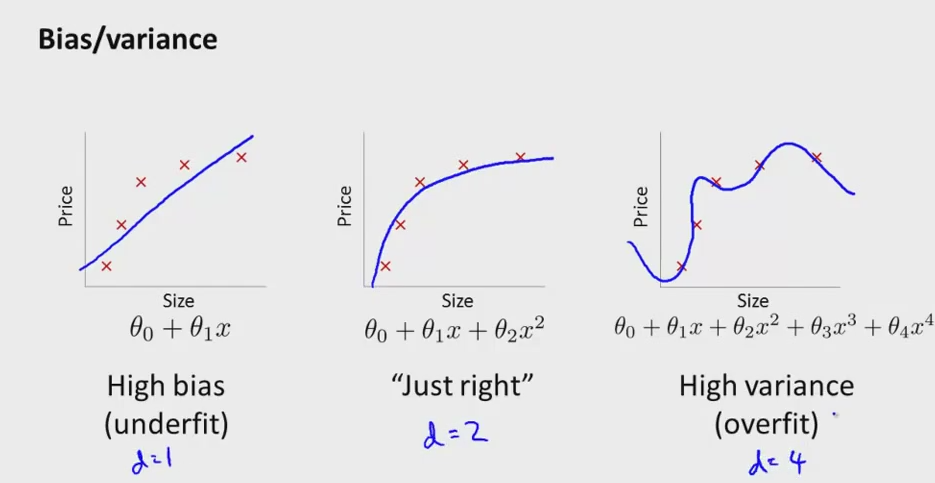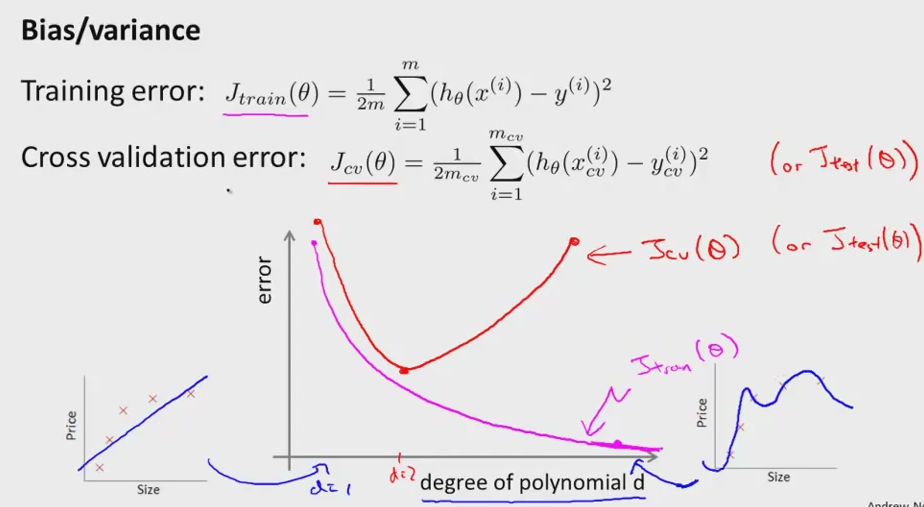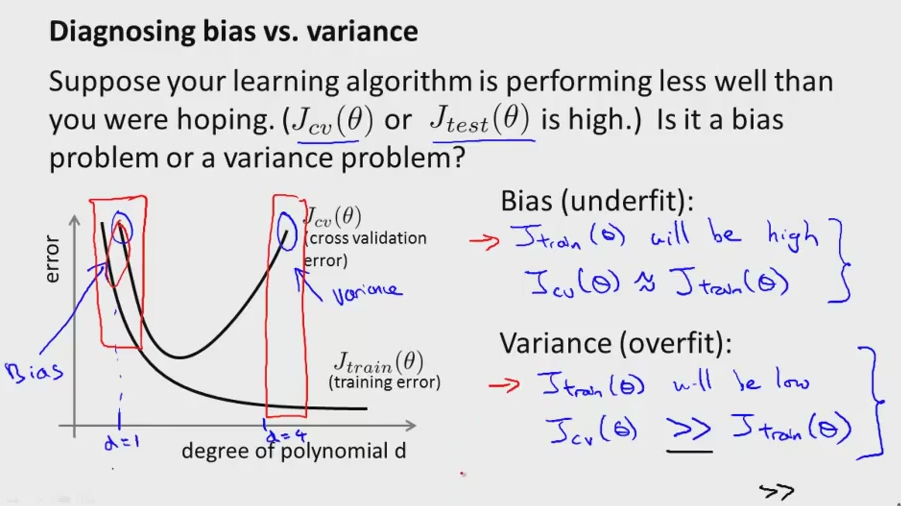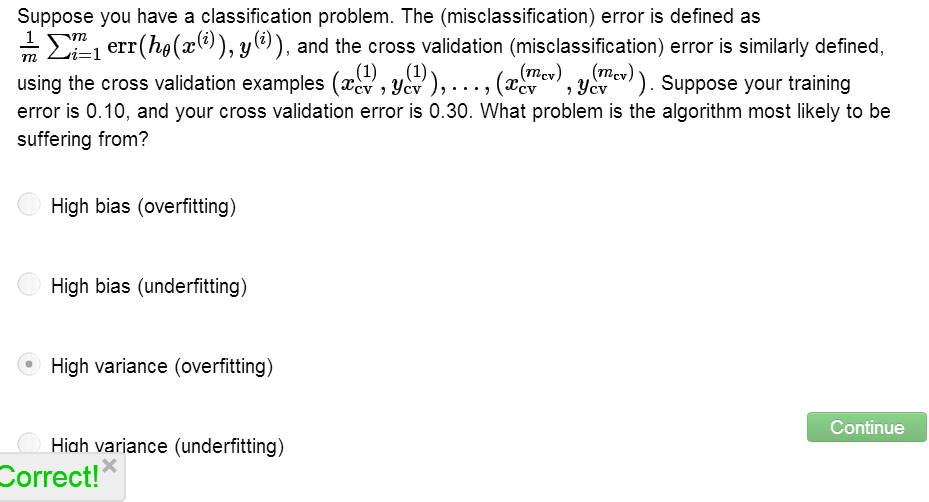• Most pitfall in machine learning is (bias)underfitting vs (variance)overfitting problems
• Knowing which happening is the important way to understand more, and fix our learning algorithms• These graph will understand bias/variance better• more and more  to the right of the diagram is the higher order polynomials (more complicated)
• Typically more high order polynomials less the error it will be
• Cross validation error should be approach the test error
• d = 2 is the better one because less will be underfitting and d = 4 will be overfitting• Learning algorightm is far from correct denotes by high point in the graph above
• But which are the bias/variance?
• left square red is the example of high bias
• right square red is the example of high variance
• To avoid underfitting or the overfitting, we must go into inside the barrier that shown from each high bias boundaries and high variance boundaries respectively• An example of high variance (overfitting) where the Jtrain(error) is very low = 0.10 , But the cross validation value much more higher = 0.3
• Occurred because the parameters much more fit to train set rathet than cross validation set.
• Later, learning algorithm in more detail from suffering of high variance/ high bias